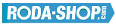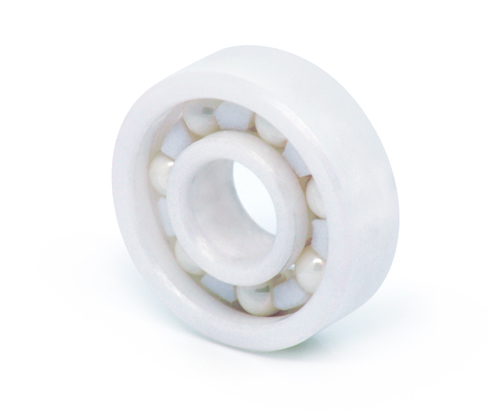# Hybrid and Ceramic BearingsManufacturer Product Image Item Name Price### 6301-CE-ZR02-PFTE

Solid Ceramic Bearings Ceramic Open(No Shields) Dimension d=12 Dimension D=37 Dimension B=12 Dinamic Load Rating=5820 Static Load Rating=2520 Weight=0.06gr. Speed limit Grease=N/A Speed limit Oil=0 Flanged
inside diameter = 12
outside diameter = 37
width = 12
call### 6302-CE-SI3N4-PFTE

Solid Ceramic Bearings Ceramic Open(No Shields) Dimension d=15 Dimension D=42 Dimension B=13 Dinamic Load Rating=6840 Static Load Rating=3270 Weight=0.083gr. Speed limit Grease=N/A Speed limit Oil=0 Flanged
inside diameter = 15
outside diameter = 42
width = 13### 6302-CE-ZR02-PFTE

Solid Ceramic Bearings Ceramic Open(No Shields) Dimension d=15 Dimension D=42 Dimension B=13 Dinamic Load Rating=6840 Static Load Rating=3270 Weight=0.083gr. Speed limit Grease=N/A Speed limit Oil=0 Flanged
inside diameter = 15
outside diameter = 42
width = 13
call### 6303-CE-SI3N4-PFTE

Solid Ceramic Bearings Ceramic Open(No Shields) Dimension d=17 Dimension D=47 Dimension B=14 Dinamic Load Rating=8160 Static Load Rating=3990 Weight=0.113gr. Speed limit Grease=N/A Speed limit Oil=0 Flanged
inside diameter = 17
outside diameter = 47
width = 14### 6303-CE-ZR02-PFTE

Solid Ceramic Bearings Ceramic Open(No Shields) Dimension d=17 Dimension D=47 Dimension B=14 Dinamic Load Rating=8160 Static Load Rating=3990 Weight=0.113gr. Speed limit Grease=N/A Speed limit Oil=0 Flanged
inside diameter = 17
outside diameter = 47
width = 14
call### 6304-CE-SI3N4-PFTE

Solid Ceramic Bearings Ceramic Open(No Shields) Dimension d=20 Dimension D=52 Dimension B=15 Dinamic Load Rating=9540 Static Load Rating=4740 Weight=0.145gr. Speed limit Grease=N/A Speed limit Oil=0 Flanged
inside diameter = 20
outside diameter = 52
width = 15### 6304-CE-ZR02-PFTE

Solid Ceramic Bearings Ceramic Open(No Shields) Dimension d=20 Dimension D=52 Dimension B=15 Dinamic Load Rating=9540 Static Load Rating=4740 Weight=0.145gr. Speed limit Grease=N/A Speed limit Oil=0 Flanged
inside diameter = 20
outside diameter = 52
width = 15
call### 6305

Solid Ceramic Bearings Ceramic Open(No Shields) Dimension d=25 Dimension D=62 Dimension B=17 Dinamic Load Rating=12360 Static Load Rating=6720 Weight=0.235gr. Speed limit Grease=N/A Speed limit Oil=0
inside diameter = 25
outside diameter = 62
width = 17### 6306

Solid Ceramic Bearings Ceramic Open(No Shields) Dimension d=30 Dimension D=72 Dimension B=19 Dinamic Load Rating=16020 Static Load Rating=9000 Weight=0.345gr. Speed limit Grease=N/A Speed limit Oil=0
inside diameter = 30
outside diameter = 72
width = 19### 6307

Solid Ceramic Bearings Ceramic Open(No Shields) Dimension d=35 Dimension D=80 Dimension B=21 Dinamic Load Rating=20100 Static Load Rating=11520 Weight=0.464gr. Speed limit Grease=N/A Speed limit Oil=0
inside diameter = 35
outside diameter = 80
width = 21